## 2.5Time derivatives

The conservation of mass Eq. (2.8 ) included a partial derivative in time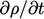relating to a ﬁxed region of space. This is the local rate of change of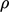, relating to the change inin the ﬂuid measured by an observer at a ﬁxed location. It is not the time rate of change experienced by a mass of ﬂuid particles as they move through space. In the same way,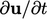is not the acceleration experienced by the ﬂuid.

Acceleration relates to the material, or substantive, derivative which describes the time rate of change of a ﬁxed mass of moving material. It is denoted by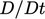and is related to the local rate of change, using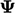as an example tensor of any rank, by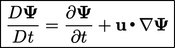(2.14)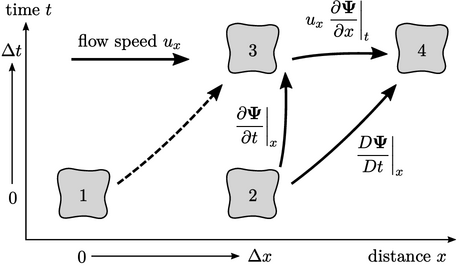The relation is derived from the chain rule of diﬀerential. In one dimension, it is illustrated by two particles of ﬂuid that occupy positions 1 and 2 at some initial time, then positions 3 and 4 at a later time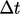. The particles move in the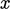-direction at speed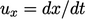, such that the particle at 1 later occupies the position 3 and the particle at 2 occupies position 4.

The material time derivative of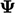, following the mass from 2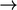4, is the sum of: the local change inat a ﬁxed position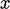(23); and, the change due to the gradient ofbetween positions 3 and 4, ﬁxing time. This equates to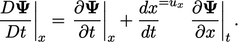(2.15)
The material derivative relation in Eq. (2.14) is simply the 3D equivalent of Eq. (2.15 ).

The last term in Eq. (2.14) introduces the gradient denoted by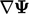. Ifis a scalar, the gradient produces a vector whose magnitude and direction is that of the steepest gradient.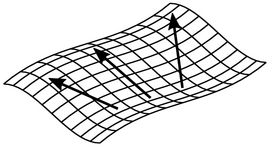When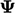is a vector, the gradient produces a tensor, representing the direction and magnitude of steepest ascent for each of the 3 components of the vector.